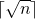# Data Structures and Algorithms | Set 13

Following questions have been asked in GATE CS 2002 exam

1. The number of leaf nodes in a rooted tree of n nodes, with each node having 0 or 3 children is:
a) n/2
b) (n-1)/3
c) (n-1)/2
d) (2n+1)/3

Let L be the number of leaf nodes and I be the number of internal nodes, then following relation holds for above given tree (For details, please see question 3 of https://www.geeksforgeeks.org/data-structures-and-algorithms-set-11/)

`  L = (3-1)I + 1 = 2I + 1`

Total number of nodes(n) is sum of leaf nodes and internal nodes

`  n = L + I `

After solving above two, we get L = (2n+1)/3

2. The running time of the following algorithm

```  Procedure A(n)
If n <= 2 return(1) else return A();
```

is best described by
a) O(n)
b) O(log n)
c) O(1og log n)
d) O(1)

For explanation, please see question 5 of https://www.geeksforgeeks.org/data-structures-and-algorithms-set-11/

3. A weight-balanced tree is a binary tree in which for each node. The number of nodes in the left sub tree is at least half and at most twice the number of nodes in the right sub tree. The maximum possible height (number of nodes on the path from the root to the farthest leaf) of such a tree on n nodes is best described by which of the following?
a) log2 n
b) log4/3 n
c) log3 n
d) log3/2 n

Let the maximum possible height of a tree with n nodes is represented by H(n).

The maximum possible value of H(n) can be approximately written using the following recursion:

```   H(n) = H(2n/3) + 1
```

The solution of above recurrence is log3/2 n. We can simply get it by drawing a recursion tree.

4. Consider the following algorithm for searching for a given number x in an unsorted – array A[1..n] having n distinct values:

```1)    Choose an i uniformly at random from 1..n;
2)    If A[i] = x then Stop else Goto 1; ```

Assuming that x is present in A, what is the expected number of comparisons made by the algorithm before it terminates?
a) n
b) n-l
c) 2n
d) n/2

If you remember the coin and dice questions, you can just guess the answer for the above.

Below is proof of the answer.

Let expected number of comparisons be E. Value of E is sum of following expression for all the possible cases.

```number_of_comparisons_for_a_case * probability_for_the_case
```

Case 1

```  If A[i] is found in the first attempt
number of comparisons = 1
probability of the case  = 1/n
```

Case 2

```  If A[i] is found in the second attempt
number of comparisons = 2
probability of the case  = (n-1)/n*1/n
```

Case 3

```  If A[i] is found in the third attempt
number of comparisons = 2
probability of the case  = (n-1)/n*(n-1)/n*1/n
```

There are actually infinite such cases. So, we have following infinite series for E.

```E  = 1/n + [(n-1)/n]*[1/n]*2 + [(n-1)/n]*[(n-1)/n]*[1/n]*3 + ….  (1)
```

After multiplying equation (1) with (n-1)/n, we get

```E (n-1)/n = [(n-1)/n]*[1/n] + [(n-1)/n]*[(n-1)/n]*[1/n]*2 +
[(n-1)/n]*[(n-1)/n]*[(n-1)/n]*[1/n]*3 ……….(2)
```

Subtracting (2) from (1), we get

```E/n = 1/n + (n-1)/n*1/n + (n-1)/n*(n-1)/n*1/n + …………
```

The expression on the right side is a GP with infinite elements. Let us apply the sum formula (a/(1-r))

```  E/n = [1/n]/[1-(n-1)/n]  = 1
E = n
```My Personal Notes arrow_drop_up

Article Tags :

Be the First to upvote.

Please write to us at contribute@geeksforgeeks.org to report any issue with the above content.